# NCERT Solutions for Class 11 Chemistry Structure of Atom Part 3## myCBSEguide App

Complete Guide for CBSE Students

NCERT Solutions, NCERT Exemplars, Revison Notes, Free Videos, CBSE Papers, MCQ Tests & more.

NCERT solutions for Class 11 Chemistry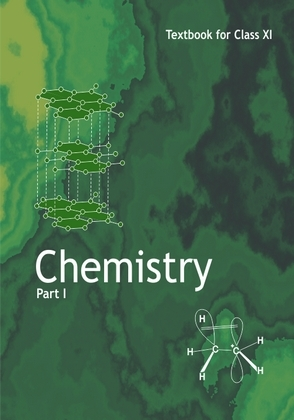## NCERT Class 11 Chemistry Chapter wise Solutions

• Chapter 1 – Some Basic Concepts of Chemistry
• Chapter 2 – Structure of Atom
• Chapter 3 – Classification of Elements and Periodicity in Properties
• Chapter 4 – Chemical Bonding and Molecular Structure
• Chapter 5 – States of Matter
• Chapter 6 – Thermodynamics
• Chapter 7 – Equilibrium
• Chapter 8 – Redox Reactions
• Chapter 9 – Hydrogen
• Chapter 10 – The s-Block Elements
• Chapter 11 – The p-Block Elements
• Chapter 12 – Organic Chemistry – Some Basic Principles and Techniques
• Chapter 13 – Hydrocarbons
• Chapter 14 – Environmental Chemistry

## NCERT Solutions for Class 11 Chemistry Structure of Atom Part 3

33. What transition in the hydrogen spectrum would have the same wavelength as the Balmer transition n = 4 to n = 2 of He+ spectrum?

34. Calculate the energy required for the process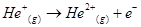The ionization energy for the H atom in the ground state is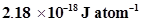35. If the diameter of a carbon atom is 0.15 nm, calculate the number of carbon atoms which can be placed side by side in a straight line across length of scale of length 20 cm long.

36.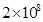atoms of carbon are arranged side by side. Calculate the radius of carbon atom if the length of this arrangement is 2.4 cm.

37. The diameter of zinc atom is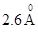.Calculate (a) radius of zinc atom in pm and (b) number of atoms present in a length of 1.6 cm if the zinc atoms are arranged side by side lengthwise.

38. A certain particle carries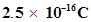of static electric charge. Calculate the number of electrons present in it.

39. In Milikan’s experiment, static electric charge on the oil drops has been obtained by shining X-rays. If the static electric charge on the oil drop is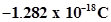, calculate the number of electrons present on it.

40. In Rutherford’s experiment, generally the thin foil of heavy atoms, like gold, platinum etc. have been used to be bombarded by the α-particles. If the thin foil of light atoms like aluminium etc. is used, what difference would be observed from the above results?

41. Symbols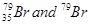can be written, whereas symbols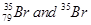are not acceptable. Answer briefly.

42. An element with mass number 81 contains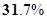more neutrons as compared to protons. Assign the atomic symbol.

43. An ion with mass number 37 possess one unit of negative charge. If the ion contains 11.1% more neutrons than electrons, find the symbol to this ion.

44. An ion with mass number 56 contains 3 units of positive charge and 30.4% more neutrons than electrons. Assign the symbol to this ion.

45. Arrange the following type of radiations in increasing order of frequency: (a) radiation from microwave oven (b) amber light from traffic signal (c) radiation from FM radio (d) cosmic rays from outer space and (e) X-rays.

46. Nitrogen laser produces a radiation at a wavelength of 337.1 nm. If the number of photons emitted is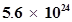, calculate the power of this laser.

47. Neon gas is generally used in the sign boards. If it emits strongly at 616 nm, calculate (a) the frequency of emission, (b) distance traveled by this radiation in 30 s (c) energy of quantum and (d) number of quanta present if it produces 2 J of energy.

48. In astronomical observations, signals observed from the distant stars are generally weak. If the photon detector receives a total of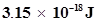from the radiations of 600 nm, calculate the number of photons received by the detector.

## NCERT Solutions for Class 11 Chemistry Chapter 2 Structure of Atom Part 3

NCERT Solutions Class 11 Chemistry Structure of Atom Part 3 PDF (Download) Free from myCBSEguide app and myCBSEguide website. Ncert solution class 11 Chemistry includes text book solutions from both part 1 and part 2. NCERT Solutions for CBSE Class 11 Chemistry have total 14 chapters. 11 Chemistry NCERT Solutions in PDF for free Download on our website. Ncert Chemistry class 11 solutions PDF and Chemistry ncert class 11 PDF solutions with latest modifications and as per the latest CBSE syllabus are only available in myCBSEguide

## NCERT Solutions for Science Class 3rd to 12th

To download NCERT Solutions for class 11 Chemistry, Physics, Biology, History, Political Science, Economics, Geography, Computer Science, Home Science, Accountancy, Business Studies and Home Science; do check myCBSEguide app or website. myCBSEguide provides sample papers with solution, test papers for chapter-wise practice, NCERT solutions, NCERT Exemplar solutions, quick revision notes for ready reference, CBSE guess papers and CBSE important question papers. Sample Paper all are made available through the best app for CBSE students and myCBSEguide.com website.## Test Generator

Create Papers with your Name & Logo